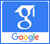# Coherent state## Quantum analysis of sub harmonic generation with two-mode coherent light

Published on: 9th April, 2021

OCLC Number/Unique Identifier: 9026720282

In this work the statistical and squeezing properties of light-driven by sub-harmonic generation with two-mode coherent light are studied. With interaction Hamiltonian of both two-mode coherent and sub harmonic generation, we have driven master equation of system under consideration. From the master equation, the solution of the C-number Langevin equation is derived. It helps us to solve quadrature variance, quadrature squeezing, mean, and variance of photon number for light produced by sub-harmonic generation with the two-mode coherent light state. And the result shows that; the squeezing occurs in plus quadrature with the maximum squeezing of 87%. The photon statistics of the system under consideration is subpoissonian in which both mean & variance are increasing as kappa increase.